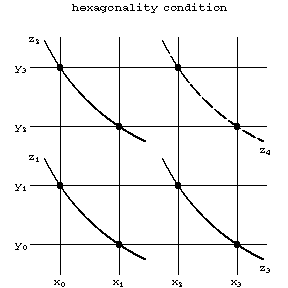# Closure condition

The condition in the geometry of webs (cf. Webs, geometry of) according to which certain incidences of points and lines of a web imply new incidences. For example, Thomsen's closure condition (see Fig.a) is as follows. The first and the second family of lines of a web are represented by parallel straight lines, the third by curves; the closure condition implies that if \$A,B,C,D\$ lie on curves of the third family, then so do \$E\$ and \$F\$.Figure: c022610a

If \$x,y\$ and \$z\$ are the parameters defining the lines of the three families, the closure condition can be presented in abstract form as a system of defining equations, regarding \$z\$ as the "product" of \$x\$ and \$y\$: \$z=xy\$,

\$\$x_0y_1=x_1y_0,x_0y_2=x_2y_0\succeq x_1y_2=x_2y_1.\$\$

If \$z=xy\$ is regarded as a quasi-group operation, Thomsen's closure condition is equivalent to the condition that the quasi-group be isotopic to an Abelian group.Figure: c022610bFigure: c022610c

Fig.b and Fig.c illustrate the Reidemeister closure condition and the hexagonality condition (all three conditions are equivalent for a plane three-web, even without a differentiability assumption). In abstract form these conditions yield different classes of quasi-groups and loops; in the multi-dimensional geometry of webs, they yield different classes of webs.

Some theorems in projective geometry are essentially closure conditions (such as the theorems of Desargues and Pappus).

How to Cite This Entry:
Closure condition. Encyclopedia of Mathematics. URL: http://encyclopediaofmath.org/index.php?title=Closure_condition&oldid=32395
This article was adapted from an original article by V.V. Ryzhkov (originator), which appeared in Encyclopedia of Mathematics - ISBN 1402006098. See original article